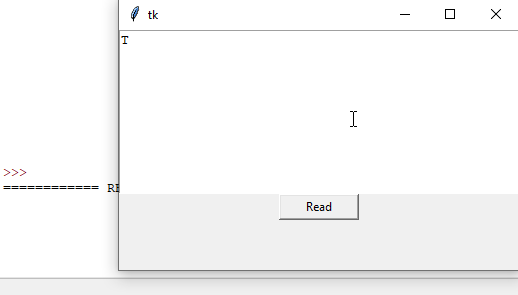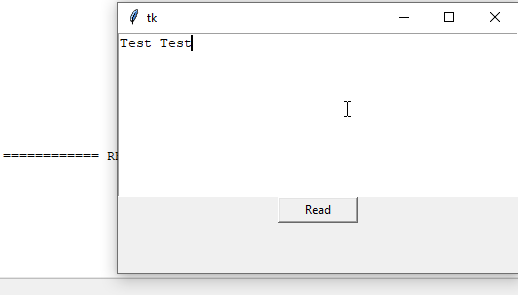# 如何獲取 Tkinter 文字框中的輸入

Tkinter `Text` 文字框控制元件具有 `get()` 方法以從文字框中返回輸入，該文字框具有 `start` 位置引數和可選的 `end` 引數來指定要獲取的文字的結束位置。

``````get(start, end=None)
``````

## 從 Tkinter 文字控制元件獲取輸入的示例程式碼

``````import tkinter as tk
root = tk.Tk()
root.geometry("400x240")

def getTextInput():
result=textExample.get("1.0","end")
print(result)

textExample=tk.Text(root, height=10)
textExample.pack()
command=getTextInput)

root.mainloop()
``````
``````result=textExample.get("1.0", "end")
``````

Tkinter 文字框控制元件中第一個字元的位置是 `1.0`，可以用數字 `1.0` 或字串`"1.0"`來表示。

`"end"`表示它將讀取直到文字框的結尾的輸入。我們也可以在這裡使用 `tk.END` 代替字串`"end"``"end-1c"`表示位置是在`"end"`之前的一個字元。

## 從 Tkinter 文字控制元件最後獲取不帶`\n` 的輸入的示例程式碼

``````import tkinter as tk
root = tk.Tk()
root.geometry("400x240")

def getTextInput():
result=textExample.get(1.0, tk.END+"-1c")
print(result)

textExample=tk.Text(root, height=10)
textExample.pack()
command=getTextInput)